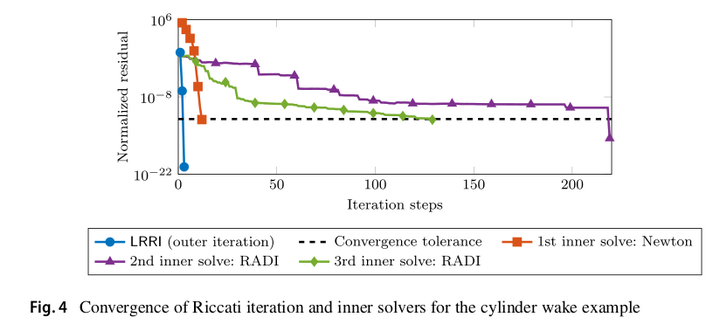# A low-rank solution method for Riccati equations with indefinite quadratic terms### Abstract

Algebraic Riccati equations with indefinite quadratic terms play an important role in applications related to robust controller design. While there are many established approaches to solve these in case of small-scale dense coefficients, there is no approach available to compute solutions in the large-scale sparse setting. In this paper, we develop an iterative method to compute low-rank approximations of stabilizing solutions of large-scale sparse continuous-time algebraic Riccati equations with indefinite quadratic terms. We test the developed approach for dense examples in comparison to other established matrix equation solvers, and investigate the applicability and performance in large-scale sparse examples.

Type
Publication
Numerical Algorithms